acousticSharpness

Perceived sharpness of acoustic signal

Description

example

sharpness = acousticSharpness(audioIn,fs) returns sharpness in acum according to DIN 45692  and ISO 532-1:2017(E) .

sharpness = acousticSharpness(audioIn,fs,calibrationFactor) specifies a nondefault microphone calibration factor used to compute loudness.

example

sharpness = acousticSharpness(SPLIn) computes sharpness using one-third-octave-band sound pressure levels (SPL).

example

sharpness = acousticSharpness(specificLoudnessIn) computes sharpness using specific loudness.

example

sharpness = acousticSharpness(___,Name,Value) specifies options using one or more Name,Value pair arguments.

Example: sharpness = acousticSharpness(audioIn,fs,calibrationFactor,'SoundField','diffuse') returns sharpness assuming a diffuse sound field.

example

acousticSharpness(___,TimeVarying,true) with no output arguments plots sharpness relative to time.

Examples

collapse all

Compute the acoustic sharpness of turbine noise. Assume it is stationary and was recorded in a diffuse sound field.

sharpness = acousticSharpness(audioIn,fs,'SoundField','diffuse');

fprintf('Acoustic sharpness = %0.2f acum\n',sharpness)
Acoustic sharpness = 1.11 acum

Plot the time-varying sharpness of the signal. Listen to the signal.

acousticSharpness(audioIn,fs,'TimeVarying',true)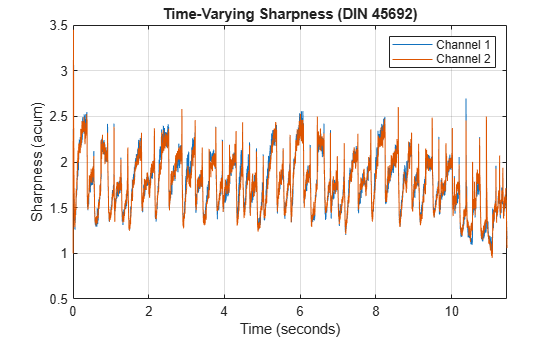sound(audioIn,fs)

Create two stationary signals with equivalent power: a pink noise signal and a white noise signal.

fs = 48e3;
dur = 5;
pnoise = 2*pinknoise(dur*fs);
wnoise = rand(dur*fs,1) - 0.5;
wnoise = wnoise*sqrt(var(pnoise)/var(wnoise));

Call acousticLoudness using the default ISO 532-1 (Zwicker) method and no output arguments to plot the loudness of the pink noise. Call acousticLoudness again, this time with output arguments, to get the specific loudness.

figure
acousticLoudness(pnoise,fs)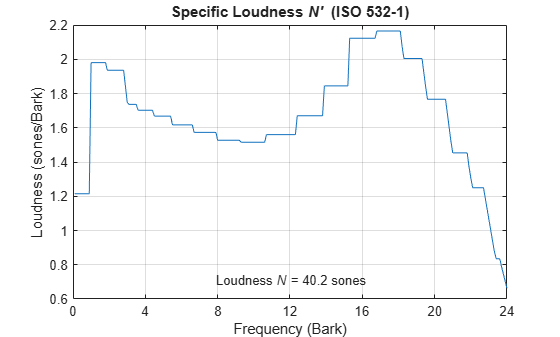[~,pSpecificLoudness] = acousticLoudness(pnoise,fs);

Plot the loudness for the white noise signal and then get the specific loudness values.

figure
acousticLoudness(wnoise,fs)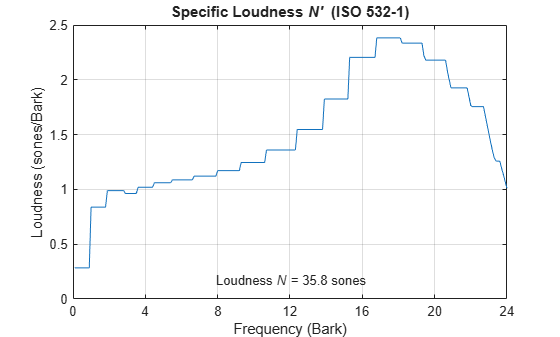[~,wSpecificLoudness] = acousticLoudness(wnoise,fs);

Call the acousticSharpness function to compare the sharpness of the pink noise and white noise.

pSharpness = acousticSharpness(pSpecificLoudness);
wSharpness = acousticSharpness(wSpecificLoudness);
fprintf('Sharpness of pink noise = %0.2f acum\n',pSharpness)
Sharpness of pink noise = 2.00 acum
fprintf('Sharpness of white noise = %0.2f acum\n',wSharpness)
Sharpness of white noise = 2.62 acum

Create a pink noise signal with a 48 kHz sample rate and a duration of 5 seconds.

fs = 48e3;
n = fs*5;
pnoise = pinknoise(n);

Specify a vector to sweep over the dB range from -60 to 20. Create a gain vector which, when multiplied by the original signal, results in a signal with the desired output level.

dBSweep = -60:10:20;
coefSweep = sqrt((10.^(dBSweep/10))/var(pnoise));

Call acousticSharpness in a loop with the different signal levels. Determine the sharpness using the default DIN 45692 frequency weighting and the Aures frequency weighting.

sharpnessDIN45692 = zeros(numel(dBSweep),1);
sharpnessAures = zeros(numel(dBSweep),1);
for ii = 1:numel(dBSweep)
signal = pnoise*coefSweep(ii);
sharpnessDIN45692(ii) = acousticSharpness(signal,fs);
sharpnessAures(ii) = acousticSharpness(signal,fs,'Weighting','Aures');
end

Display the effect of the input level on the acoustic sharpness. The Aures frequency weighting method is more sensitive to the input level.

plot(dBSweep,sharpnessDIN45692,dBSweep,sharpnessAures)
legend('Weighting = DIN45692','Weighting = Aures')
xlabel('Input Level (dB)')
ylabel('Sharpness (acum)')
title('Effect of Input Level on Sharpness')
axis([dBSweep(1) dBSweep(end) 0 20])
grid on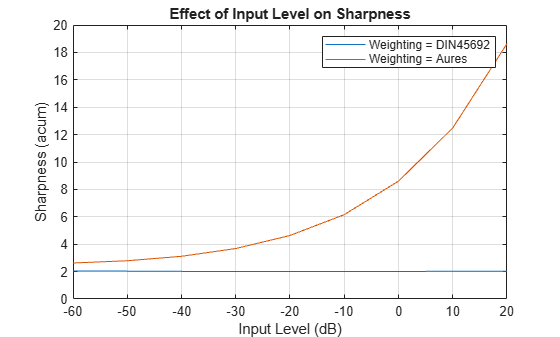Read in two audio files: one of an electric guitar with distortion and one of an acoustic guitar. Both audio files have a sample rate of 44.1 kHz. For easy comparison, convert the rock guitar signal to mono and shorten the soft guitar signal to the length of the rock guitar signal.

fs = 44.1e3;
rockGuitar = mean(rockGuitar,2);
softGuitar = softGuitar(1:numel(rockGuitar));

Calculate the time-varying sharpness for both the rock guitar and soft guitar.

rGSharpness = acousticSharpness(rockGuitar,fs,'TimeVarying',true);
sGSharpness = acousticSharpness(softGuitar,fs,'TimeVarying',true);

Plot the probability distribution based on the observed sharpness of the rock guitar and the soft guitar.

histogram(rGSharpness,'Normalization','probability')
hold on
histogram(sGSharpness,'Normalization','probability')
legend('Electric Guitar','Soft Guitar')
xlabel('Sharpness (acum)')
ylabel('Probability')
title('Time-Varying Acoustic Sharpness (DIN 45692)')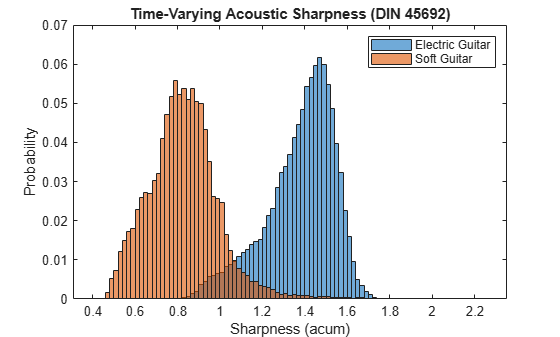To calculate sound pressure levels from an audio signal, first create an splMeter object. Call the splMeter object with the audio input.

spl = splMeter("SampleRate",fs,"Bandwidth","1/3 octave", ...
"FrequencyWeighting","Z-weighting","OctaveFilterOrder",6);

splMeasurement = spl(audioIn);

Compute the mean SPL level, skipping the first 0.2 seconds. Only keep the bands from 25 Hz to 12.5 kHz (the first 28 bands).

SPLIn = mean(splMeasurement(ceil(0.2*fs):end,1:28));

To determine the acoustic sharpness of the audio signal, call acousticSharpness using the sound pressure level input.

sharpness = acousticSharpness(SPLIn)
sharpness = 1.1020

Input Arguments

collapse all

Audio input, specified as a column vector (mono) or matrix with two columns (stereo). Sharpness is computed for each channel (column) independently.

Data Types: single | double

Sample rate in Hz, specified as a positive scalar. The recommended sample rate for new recordings is 48 kHz.

Note

The minimum acceptable sample rate is 8 kHz.

Data Types: single | double

Microphone calibration factor, specified as a positive scalar. The default calibration factor corresponds to a full-scale 1 kHz sine wave with a sound pressure level of 100 dB (SPL). To compute the calibration factor specific to your system, use the calibrateMicrophone function.

Data Types: single | double

Sound pressure level (SPL) in dB, specified as a 1-by-28-by-C array. 28 corresponds to one-third-octave bands between 25 Hz and 12.5 kHz. C is the number of channels.

Data Types: single | double

Specific loudness in sones/Bark, specified as a T-by-240-by-C array, where:

• T is 1 for stationary signals or one per 2 ms for time-varying signals.

• 240 is the number of Bark bins in the domain for specific loudness. The Bark bins are 0.1:0.1:24.

• C is the number of channels.

You can use the acousticLoudness function to calculate specificLoudnessIn using this syntax:

[~,specificLoudnessIn] = acousticLoudness(audioIn,fs);

Data Types: single | double

Name-Value Arguments

Specify optional comma-separated pairs of Name,Value arguments. Name is the argument name and Value is the corresponding value. Name must appear inside quotes. You can specify several name and value pair arguments in any order as Name1,Value1,...,NameN,ValueN.

Example: acousticSharpness(audioIn,fs,'Weighting','von Bismarck')

Frequency weighting, specified as 'DIN 45692', 'Aures', or 'von Bismarck'. By design, the 'Aures' frequency weighting method is more sensitive to amplitude levels and proper calibration. For details, see Algorithms.

Data Types: char | string

Sound field of audio recording, specified as 'free' or 'diffuse'.

Data Types: char | string

Reference pressure for dB calculation in pascals, specified as a positive scalar. The default value, 20 micropascals, is the common value for air.

Data Types: single | double

Input is time-varying, specified as true or false. If TimeVarying is set to true, acoustic sharpness is calculated in 2 ms intervals.

Data Types: logical

Output Arguments

collapse all

Acoustic sharpness in acum, returned as a scalar, vector, or matrix. Sharpness is computed according to DIN 45692 and ISO 532-1.

Data Types: single | double

Algorithms

Acoustic sharpness is a measurement derived from acoustic loudness. The acoustic loudness algorithm is described in  and implemented in the acousticLoudness function. The acoustic sharpness calculation is described in . The algorithm for acoustic sharpness is outlined as follows.

$sharpness\text{\hspace{0.17em}}=\text{\hspace{0.17em}}k\text{\hspace{0.17em}}\left(\frac{\underset{z=0}{\overset{24}{\int }}N\text{'}\left(z\right)\text{\hspace{0.17em}}g\left(z\right)\text{\hspace{0.17em}}z\text{\hspace{0.17em}}\text{d}z}{\underset{z=0}{\overset{24}{\int }}N\text{'}\left(z\right)\text{\hspace{0.17em}}\text{d}z}\right)$

Where N' is the specific loudness in sones/Bark. The function g(z) and the scaling factor k depend on the specified Weighting method:

'DIN 45692': k is set such that a 1 kHz reference tone results in a 1 acum sharpness measurement, and

$\begin{array}{lll}g\left(z\right)=1\hfill & \text{for}\hfill & z\le 15.8\text{\hspace{0.17em}}\text{Bark}\hfill \\ g\left(z\right)=0.15{e}^{0.42\left(z-15.8\right)}+0.85\hfill & \text{for}\hfill & z>15.8\text{​}\text{\hspace{0.17em}}\text{Bark}\hfill \end{array}$

'von Bismark': k is set to 0.11, and

$\begin{array}{lll}g\left(z\right)=1\hfill & \text{for}\hfill & z\le 15\text{\hspace{0.17em}}\text{Bark}\hfill \\ g\left(z\right)=0.2{e}^{0.308\left(z-15\right)}+0.8\hfill & \text{for}\hfill & z>15\text{​}\text{\hspace{0.17em}}\text{Bark}\hfill \end{array}$

'Aures': k is set to 0.11, and

$\begin{array}{l}g\left(z\right)=0.078\left(\frac{{e}^{0.171z}}{z}\right)\left(\frac{N}{\mathrm{ln}\left(0.05N+1\right)}\right)\\ \text{where}\\ N=\underset{z=0}{\overset{24}{\int }}N\text{'}\left(z\right)\text{\hspace{0.17em}}\text{d}z\end{array}$

 ISO 532-1:2017(E). "Acoustics – Methods for calculating loudness – Part 1: Zwicker method." International Organization for Standardization.

 DIN 45692:2009. "Measurement Technique for the Simulation of the Auditory Sensation of Sharpness." German Institute for Standardization.

Audio Toolbox Documentation